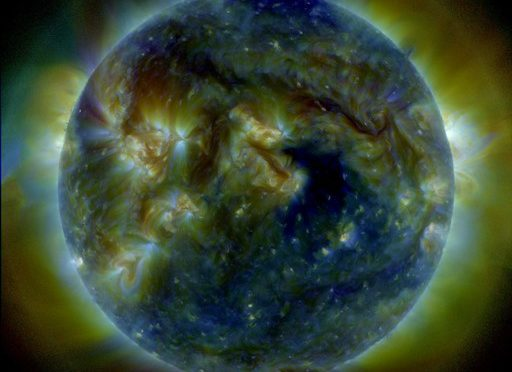# Solar wind predictionI’m going to do an experiment of predicting the solar wind speed near the Earth based on the series of the Sun images.

Skobeltsyn Institute of Nuclear Physics of Moscow State University publishes observation and prediction data on space weather. Solar wind prediction is also published there. My experiment is to try to build more accurate prediction based on the same initial data from SINP MSU.

The experiment is the following:

1. Take the data from SINP MSU
2. Prepare features data to be used as predictors
3. Prepare observational data to be used as reference values
4. Calculate error rates for current SINP MSU model
5. Designing the computational model for the solar wind
6. Fit Pulse-based model of solar wind, calculate error rates
7. Fit machine learining regression
8. Compare the error rates of each of the models

For each of this experiment phases I will publish a separate post.